Courses

# Radiation (Part - 1) Electrical Engineering (EE) Notes | EduRev

## Electrical Engineering (EE) : Radiation (Part - 1) Electrical Engineering (EE) Notes | EduRev

The document Radiation (Part - 1) Electrical Engineering (EE) Notes | EduRev is a part of the Electrical Engineering (EE) Course Electromagnetic Theory.
All you need of Electrical Engineering (EE) at this link: Electrical Engineering (EE)

In the following two lectures we will discuss the production of electromagnetic waves. Once produced they carry their energy and momentum and propagate in free space, may be to be received by an antenna. An antenna is a physical device which transmits or receives electromagnetic waves.

What causes a source to emit radiation? We have seen that charges at rest creates electrostatic field. A steady current, on the other hand is a source of magnetic field. Neither of these lead to radiation. However, it is known that accelerating charges emit radiation.

To discuss this in some detail, we fall back on our workhorse, viz., the Maxwell’s equations. Let us recall the potential formulation of the electromagnetic field that we had discussed earlier.

Using the expression for Faraday’s law,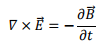We rewrite this in terms of vector potential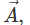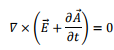This enablesus to express the quantity within the bracket as gradient of a scalar function V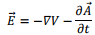Using Lorentz gauge condition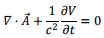the Maxwell’s equations can be expressed as a pair of homogeneous wave equations,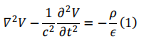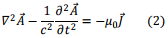Since the structure of both equations is same, we can solve either of them and write the solution to the remaining equation by similarity. We will solve the equation for the vector potential. The method of solution depends on defining a “Green’s function”.

Let us define a time Fourier transform through the equation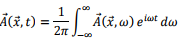the inverse of which is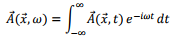Substituting in Eqn. (2)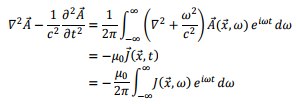Taking inverse Fourier transform for both sides, we get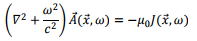Defining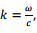we get,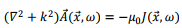To obtain the solution of this equation, we define a subsidiary equation for a function known as the Green’s function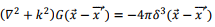Thus the Green’s function satisfies a very similar equation in which the inhomogeneous term of the original equation has been replaced by a delta function. In terms of the Green’s function the formal solution of the equation for the vector potential can be written as a convolution,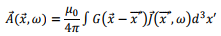To see how it comes about,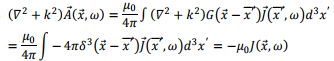Remember that in the above, the primed coordinate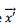is for the source and the unprimed quantity refers to the point where the field is being evaluated. The solution must be spherically symmetric about the source point, i.e. depend on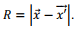With this substitution, thee quation for the Green’s function becomes,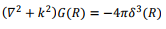First consider R ≠ 0, for which the equation becomes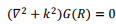Expressing the Laplacian in spherical coordinates, as there is no dependence on angles,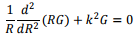the solution for which is seen to be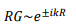so that the Green’s function, excepting at the origin has the solution as a linear combination of an outgoing wave and an incoming wave.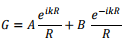What about the solution at the origin?

Let us take a small sphere of radius R0 around the origin. Since the argument of the delta function is included within the sphere, we have,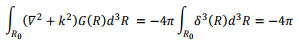Since G(R) is singular only at the origin, we can calculate the integral over it within the sphere, by replacing it by the expression obtained for it except at the origin,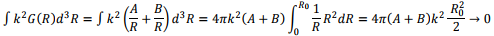asR→ 0

Thus,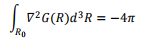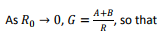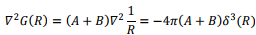Substituting this into the preceding integral, we get

A+B=1

Thus we have,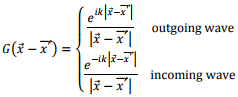We will take the outgoing solution and proceed further.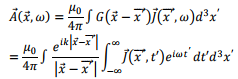Let us take the inverse Fourier transform of the above to get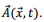It may, however, be remembered that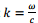has ω dependence,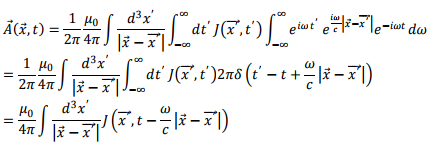Parallely, one can write, for the scalar potential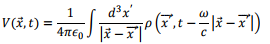Note that the potential at time t at the position where it is being calculated is given in terms of current in the source at an “earlier time”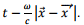The potential is influenced by the wave which came from the source at a time which is earlier by the time taken by the electromagnetic wave to travel this distance. This is known as the “retarded” potential. The other solution is the incoming wave solution which would give to an “advanced” solution.

Example : Localized oscillation of charge or current

Let us consider a localized source of charge or current, given by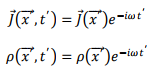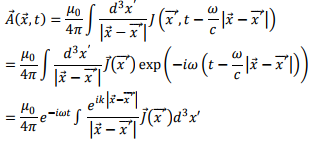Thus we have,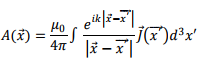and, similarly,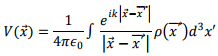where k=ω/c

There are three regions of interest, assuming that the source is confined to a small region of space with typical dimension d. The distance at which the field is being measured is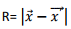and the wavelength of the radiation is λ.

1. Near field for which d <<R<<λ
2. Intermediate zone for which d <<R<<λ
3. Radiation zone or Far field with d <<λ<<R

Our primary interest will be in the radiation zone, which we will discuss in detail in the next lecture. We will have very little to say about the intermediate zone and its analysis is very complicated.

Consider the near field for which d <<R<<λ In this region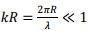as a result of which we can replace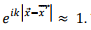The expression for the vector potential becomes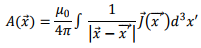which is the familiar expression for the vector potential in magnetostatics. The only difference from the magnetostatic case is the time variation of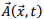which is simply to multiply the expressions obtained in the static case by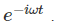Thus we can say that the situation is “quasi-static” and we can borrow all our results from magnetostatics to discuss this region.

Tutorial Assignment

1. Consider a thought experiment in which a charge q appears abruptly at the origin at time t=t1 and disappears equally abruptly at time t=tFind the retarded potentials for the problem.Determine the corresponding fields.
2. . A long straight wire lying along the z axis carries a current given by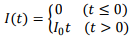Determine the retarded potentials and the electric and the magnetic fields.

Solutions to Tutorial Assignments

1. Since the charge is static only retarded scalar potential is generated. For time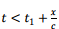the information that a charge has appeared at the origin has not reached the position x. Thus the potential is zero for such time. Likewise, though the charge has disappeared at time t, this information would not reach the position x till a later time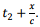Thus the potential is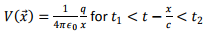and zero at other times. The corresponding field is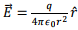in the same time interval and is zero at other times.

2. The wire being charge neutral, the scalar potential is zero. The vector potential, because of cylindrical symmetry depends only on the distance s of the point of observation P from the wire, and is given by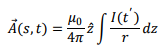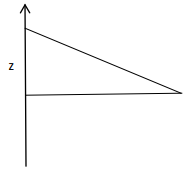Consider an element dz at a distance z form the origin (see figure). Information about the current element would reach the point P at a time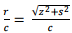later than the time the current was actually established. Thus the current, as seen by the point P is given by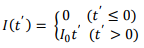where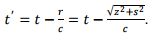Now let us look at the range of integration. For an element dz located at a distance z from the origin to influence the field at the point P, its distance from P should be less than ct. Thus,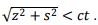Hence the potential is given by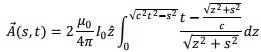(the factor of 2 in front is because only the upper half of the wire is considered in the integration). The integral is straightforward and gives,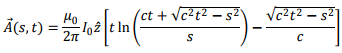The electric field is given by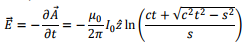and the magnetic field is given by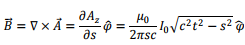Self Assessment Questions

1. An long straight wire lies along the z direction. At time t= 0, a constant current |0 is switched on. Calculate the vector potential and the electric and the magnetic fields.
2. A conducting wire is bent into a loop of two semicircles, one of radius R1 and the other R2, (<R1)connected by straight segments. The loop carries a current |(t) = |0t, which is directed anticlockwise on the shorter semicircle and clockwise on the other one.. Calculate the vector potential at the centre of the loop. Calculate the electric field at the centre. Can you calculate the magnetic field from the expression of the vector potential?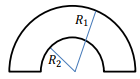Solutions to Self Assessment Questions

1.The problem is very similar to Problem 2 of the Tutorial. The vector potential is given by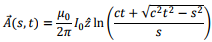2.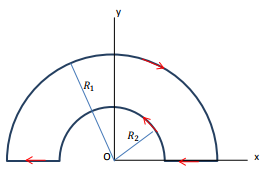Since the current is switched on at t=0, the (retarded) vector potential at the centre O is given by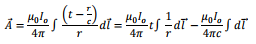Since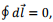the second integral vanishes. We are left with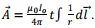Note that the integral is a line integral over the loop, which is directed differently at different points of the loop. Let us take the loop to be in the x-y plane with directions as shown in the figure. Recall that the direction of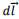is along the direction of the current. The contribution from the two straight sections give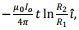the negative sign is due to the fact that the current in both the sections are directed along the negative x direction.

We now have to perform the integration over the two semicircles. Consider the integration over the bigger semicircle. We can resolve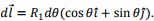The denominator of the integral also has R1 so that the integral becomes independent of R1 (In a similar way, the integral of the smaller semicircle will be independent of R2). The y-component of the integral would vanish by symmetrically placed elements on either side of the y-axis, leaving us with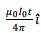as the value of the integral. The integral over the smaller semicircle is done in a similar way but its direction would be opposite because of the direction of the current. Thus the contribution from the two semicircles would cancel and the vector potential at the centre would be given by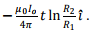The electric field is trivial and is given by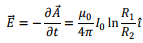Since the vector potential has been calculated only at a single point, it is not possible to calculate its curl and hence the magnetic field cannot be determined by the above calculation.

Offer running on EduRev: Apply code STAYHOME200 to get INR 200 off on our premium plan EduRev Infinity!

,

,

,

,

,

,

,

,

,

,

,

,

,

,

,

,

,

,

,

,

,

;